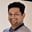Related Tags

twosum
java
hashset
communitycreator

# How can we implement the two sum problem in Java?Tarun Telang

## Problem

Given an array of unique integers, numbers, and an integer targetSum, return two numbers from the array so that they add up to targetSum. If there are no pairs with the required targetSum in the input array, then return null.

For example, for array input [3, 6, 10, 14] and targetSum = 9, it should return [3, 6] or [6, 3].

If the array is null or the length is less than two, it should return null.

In the case of an array where a pair with a sum equal to targetSum does not exist, it should return null.

## Brute force solution

Check the sums of all possible pairs in the array to see whether their sum equals the targetSum, and return the pair that satisfies this condition.

Below is the Java code for this approach.

### Code

import java.util.*;
class Solution {
// time complexity O(n^2) | space complexity O(1)
public static int[] twoSum(int[] numbers, int targetSum) {
if (numbers == null || numbers.length < 2) {
return null;
}

for (int i = 0; i < numbers.length; i++) {
int diff = targetSum - numbers[i];
for (int j = i + 1; j < numbers.length; j++) {
if (numbers[j] == diff) {
return new int[]{numbers[i], numbers[j]};
}
}
}
return null;
}

public static void main(String[] args){
System.out.println(Arrays.toString(
twoSum(new int[]{3, 6, 10, 14}, 9))); // [6, 3] best case

System.out.println(Arrays.toString(
twoSum(null, 4))); // null - invalid input

System.out.println(Arrays.toString(
twoSum(new int[]{3, 1, 4}, 9))); // null - no pair exists

System.out.println(Arrays.toString(
twoSum(new int[]{3}, 9))); // null - array with less than two integers
}
}
Brute force solution to the two sum problem

### Analysis

The code above runs in O($n{^2}$) time complexity, where n is the length of the array.

The space complexity for the code above is O(1), as we don’t need any memory other than the loops, variables, and the resultwhich is an array of size two.

## Optimal solution

We can further optimize the above solution approach by using a set.

The algorithm for this optimal approach is as follows:

1. Initialize an empty HashSet.

2. For each integer in the array:

2.1 Calculate the difference between the current integer and the targetSum.

2.2 Check whether the difference calculated above is present in the set.

• If the difference already exists in the set, return the current element and difference as the result.
• Otherwise, insert the current integer into the set.

See the visual representation below to better understand this algorithm.

Step 1
1 of 5

The Java code for this approach is below.

### Code

import java.util.*;

class Solution {
// time complexity O(n) | space complexity O(n)
public static int[] twoSum(int[] numbers, int targetSum) {
int[] result = null;

if (numbers == null || numbers.length < 2) {
return null;
}

Set<Integer> map = new HashSet<>();
for (int i= 0; i < numbers.length; i++) {
int diff = targetSum - numbers[i];
if (map.contains(diff)) {
result = new int;
result = numbers[i];
result = diff;
break;
}
}

return result;
}

public static void main(String[] args){
System.out.println(Arrays.toString(
twoSum(new int[]{3, 6, 10, 14}, 9))); // [6, 3] best case

System.out.println(Arrays.toString(
twoSum(null, 4))); // null - invalid input

System.out.println(Arrays.toString(
twoSum(new int[]{3, 1, 4}, 9))); // null - no pair exists

System.out.println(Arrays.toString(
twoSum(new int[]{3}, 9))); // null - array with less than two integers
}
}
Optimal solution for the two sum problem

### Analysis

The time complexity of the code above is O(n), where n is the length of the array. This is because we need to iterate the array only once.

The space complexity for this approach is also O(n), as we need a set whose size is dependent on n, the length of the array.

RELATED TAGS

twosum
java
hashset
communitycreator

CONTRIBUTORTarun Telang
RELATED COURSES

View all Courses

Keep Exploring

Learn in-demand tech skills in half the time